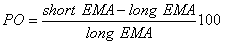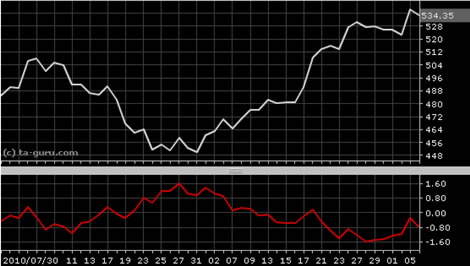Chart Pro online
User name (email):

 Recently used

 Forex

 Indices

# Price Oscillator (PO)

Price Oscillator (PO) is momentum oscillator in technical analysis which shows difference between two moving averages.

Price oscillator is calculated by formula:Price oscillator is based on exponential moving average. For short EMA period is usually used value of 9 and for long EMA value of 26.Price Oscillator (PO)

Interpretation

Generally, buying and selling signals could be created using zero line crossovers: buy signal when PO crosses above zero line and sell signal when PO crosses below zero line.

Example